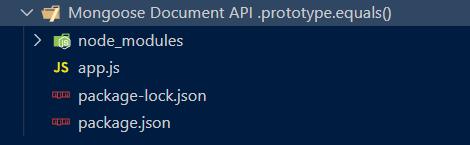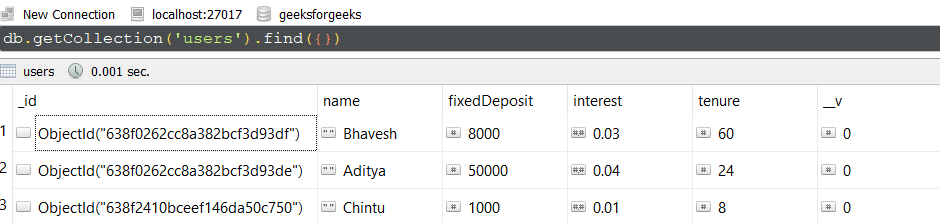Open in App
Not now

# Mongoose Document.prototype.equals() API

• Last Updated : 09 Dec, 2022

The Document API.prototype.equals() method of the Mongoose API is used on the Document Model object. It allows us to verify whether two document objects are equal or not. This method will return true if one document is equal to another document. This method uses the _id  field of documents to compare. Let us understand equals() method using an example.

Syntax:

`document1.equals( document2 );`

Parameters: This method accepts a single parameter as described below:

• document2: It is used to specify the second document that needs to be compared with the first one.

Return Value: This method returns Boolean value. If both the documents are equal to each other method will return true else false.

Setting up Node.js Mongoose Module:

Step 1: Create a Node.js application using the following command:

`npm init`

Step 2: After creating the NodeJS application, Install the required module using the following command:

`npm install mongoose`

Project Structure: The project structure will look like this:Database Structure: The database structure will look like this, the following documents are present in the collection.Example 1: In this example, we have established a database connection using mongoose and defined model over userSchema, having five columns or fields “_id”, “name”, “fixedDeposit”, “interest”, and “tenure”. We have used find() method which return array of all documents. Using indexing we are extracting first two documents and comparing both the documents using equals() method.

Filename: app.js

## Javascript

 `// Require mongoose module ` `const mongoose = require(``"mongoose"``); ` ` `  `// Set Up the Database connection ` `mongoose.connect(``"mongodb://localhost:27017/geeksforgeeks"``, { ` `    ``useNewUrlParser: ``true``, ` `    ``useUnifiedTopology: ``true``, ` `}); ` ` `  `const userSchema = ``new` `mongoose.Schema({ ` `    ``name: String, ` `    ``fixedDeposit: Number, ` `    ``interest: Number, ` `    ``tenure: Number ` `}); ` ` `  `const User = mongoose.model(``'User'``, userSchema); ` ` `  `const equalsExample1 = async () => { ` `    ``const documents = await User.find(); ` `    ``const document1 = documents; ` `    ``const document2 = documents; ` `    ``const equalOrNot = document1.equals(document2); ` `    ``console.log(equalOrNot) ` `} ` ` `  `equalsExample1();`

Step to run the program: To run the application execute the below command from the root directory of the project:

`node app.js`

Output:

`false`

Example 2: In this example, we have established a database connection using mongoose and defined model over userSchema, having five columns or fields “_id”, “name”, “fixedDeposit”, “interest”, and “tenure”. At the end, we are comparing same document to see the true as returned value as equals() method compare’s _id field.

## Javascript

 `// Require mongoose module ` `const mongoose = require(``"mongoose"``); ` ` `  `// Set Up the Database connection ` `mongoose.connect(``"mongodb://localhost:27017/geeksforgeeks"``, { ` `    ``useNewUrlParser: ``true``, ` `    ``useUnifiedTopology: ``true``, ` `}); ` ` `  `const userSchema = ``new` `mongoose.Schema({ ` `    ``name: String, ` `    ``fixedDeposit: Number, ` `    ``interest: Number, ` `    ``tenure: Number ` `}); ` ` `  `const User = mongoose.model(``'User'``, userSchema); ` ` `  `User.findOne().then(document1 => { ` `    ``console.log(document1.equals(document1)); ` `});`

Step to run the program: To run the application execute the below command from the root directory of the project:

`node app.js`

Output:

`true`

My Personal Notes arrow_drop_up
Related Articles